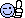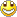Welcome GuestYou last visited September 23, 2020, 1:09 am
All times shown are
Eastern Time (GMT-5:00)

# Are correct probability calculation?

Topic closed. 7 replies. Last post 6 years ago by SergeM.

 Page 1 of 1
 febausaThread StarterNew MemberCoral SpringsUnited StatesMember #153336March 13, 201419 PostsOffline March 13, 2014, 4:35 pm⮟Are correct this calculation? , Please recommend it correct calculationRegular ball a total of 75 numbersThe probability of select the first number in the Mega million = 1/75The probability of select the second number in the Mega million = 1/74The probability of select the third number in the Mega million = 1/73The probability of select the fourth number in the Mega million = 1/72The probability of select the fifth number in the Mega million = 1/71The selected numbers or winning numbers are not replaced in the basket, then the probability of choosing five numerous is the sum of the prior probabilities = 1/75 + 1/74 + 1/73 + 1/72 + 1/71 =
 LottoMetroHappylandUnited StatesMember #146340September 1, 20131146 PostsOffline March 13, 2014, 4:55 pmQuote: Originally posted by febausa on March 13, 2014Are correct this calculation? , Please recommend it correct calculationRegular ball a total of 75 numbersThe probability of select the first number in the Mega million = 1/75The probability of select the second number in the Mega million = 1/74The probability of select the third number in the Mega million = 1/73The probability of select the fourth number in the Mega million = 1/72The probability of select the fifth number in the Mega million = 1/71The selected numbers or winning numbers are not replaced in the basket, then the probability of choosing five numerous is the sum of the prior probabilities = 1/75 + 1/74 + 1/73 + 1/72 + 1/71 =1) Close, but you don't add. You multiply and get probability = 4.82829E-102) Divide 1/probability found in #1 = 2,071,126,8003) Find 5! (factorial 5, or 5*4*3*2*1) since you can choose the 5 numbers in any order = 1204) Divide #2 by #3 = 17,259,3905) For MM, multiply #4 by 15 since there are 15 different Mega balls = 258,890,850Those are the odds. You can divide 1 by 258,890,850 to get the probability in decimal form. Note that if you compare #4 to the odds for 2nd prize in MM, it will be different. This is because their odds are the odds of matching only that prize and no other prize (which is harder). To get these odds you would multiply #4 by 15/14 to get approximately 18,492,204.If the chances of winning the jackpot are so slim, why play when the jackpot is so small? Your chances never change, but the potential payoff does. If a crystal ball showed you the future of the rest of your life, and in that future you will never win a jackpot, would you still play?P&L % = Total Win(\$)/Total Wager(\$) - 1
 LottoMetroHappylandUnited StatesMember #146340September 1, 20131146 PostsOffline March 13, 2014, 5:21 pmBy the way, a shortcut to bypass the probability conversion would be:= 75*74*73*72*71 / 5!That gives you #4, then just multiply by 15 to get #5, which you can divide into 1 to get the actual probability. Even easier would be to use Excel's COMBIN function or TI-84 calculator nCr function to get "n choose k," where n = 75 and k = 5. Then do step #5 as prescribed.If the chances of winning the jackpot are so slim, why play when the jackpot is so small? Your chances never change, but the potential payoff does. If a crystal ball showed you the future of the rest of your life, and in that future you will never win a jackpot, would you still play?P&L % = Total Win(\$)/Total Wager(\$) - 1Economy class
Belgium
Member #123696
February 27, 2012
4035 Posts
Offline
 5 1 4 350 3 24150 2 547400 1 4584475 0 12103014New Member
Coral Springs
United States
Member #153336
March 13, 2014
19 Posts
Offline
 5 1 4 350 3 24150 2 547400 1 4584475 0 12103014

 SergeMEconomy classBelgiumMember #123696February 27, 20124035 PostsOffline March 14, 2014, 7:03 pmQuote: Originally posted by febausa on March 14, 2014Please explain more your comment. I don't understand.5/5 from 75 numbers : 1 time 4/5 ...
 febausaThread StarterNew MemberCoral SpringsUnited StatesMember #153336March 13, 201419 PostsOffline March 16, 2014, 6:45 pmVery good answer, thank you
 SergeMEconomy classBelgiumMember #123696February 27, 20124035 PostsOffline March 25, 2014, 1:04 pmI thought you might figure that one out on your own.